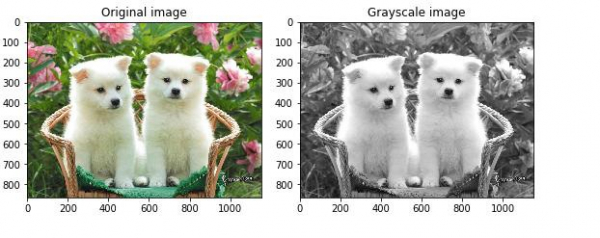# How can scikit-learn be used to convert an image from RGB to grayscale in Python?

Scikit-learn, commonly known as sklearn is a library in Python that is used for the purpose of implementing machine learning algorithms.

Conversion of an image from one color space to another is usually used so that the newly achieved color space can prove as a better input to perform other operations on it. This includes separating hues, luminosity, saturation levels, and so on. When an image is represented using RGB representation, the hue and luminosity attributes are shown as a linear combination of channels R, G and B.

When an image with RGB color space is tried to be converted to grayscale, the pixel values are calculated as the weighted sum of the red, green and blue pixels. The equation has been shown below −

Y = 0.2125 R + 0.7154 G + 0.0721 B

These weights are specifically provided, since they are used by CRT phosphors that represent human perception of the colors red, green and blue better, in comparison to providing equal weights for all the three values.

Let us see how an RGB image can be converted into a grayscale image −

## Example

from skimage import io
import matplotlib.pyplot as plt
from skimage import data
from skimage.color import rgb2gray
from skimage import data
path = "path to puppy_1.JPG"
grayscale_img = rgb2gray(orig_img)
fig, axes = plt.subplots(1, 2, figsize=(8, 4))
ax = axes.ravel()
ax.imshow(orig_img)
ax.set_title("Original image")
ax.imshow(grayscale_img, cmap=plt.cm.gray)
ax.set_title("Grayscale image")
fig.tight_layout()
plt.show()

## Output## Explanation

• The required libraries are imported.
• The path where the image is stored is defined.
• The ‘imread’ function is used to visit the path and read the image.
• The ‘imshow’ function is used to display the image on the console.
• The function ‘rgb2gray’ is used to convert the image from RGB color space to grayscale color space.
• The matplotlib library is used to plot this data, and show the original image and the image after being converted to grayscale.
• This is displayed on the console.

Updated on: 11-Dec-2020

1K+ Views